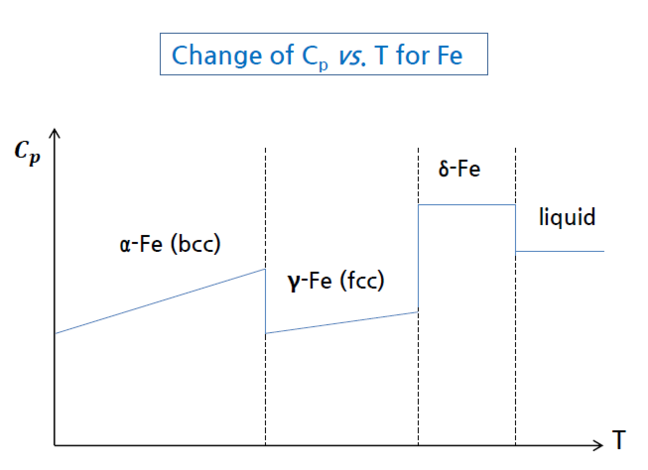## Want to keep learning?

This content is taken from the Hanyang University's online course, Thermodynamics in Energy Engineering. Join the course to learn more.
1.13

# T_ P dependence of heat capacity

Heat capacity

#### ♦  Temperature dependence of heat capacity

• $$C$$$$p$$ usually give as $$C$$$$p$$=$$a$$+$$bT$$+$$cT$$−$$2$$+$$dT$$$$2$$
• When phase changes → Heat capacity changes abruptly#### ♦  Pressure dependence of heat capacity

• $$C$$$$p$$ of ideal gas
For ideal gas, $$C$$$$p$$ is independence of pressure. Each molecule behaves        independently.

• $$C$$$$p$$ of solid
In most cases, $$C$$$$p$$ is a function of temperature and not a function of        pressure, but at high pressire, $$C$$$$p$$ is strongly dependent on pressure.